﻿ An Algebraic Derivation of Buffer Capacity

### An Algebraic Derivation of Buffer Capacity

Matthew S. Fox, Joshua W. Greenberg, Jake M. Trookman

World Journal of Chemical Education

## An Algebraic Derivation of Buffer Capacity

Matthew S. Fox1,, Joshua W. Greenberg1, Jake M. Trookman1

1Department of Chemistry, Cheyenne Mountain High School, Colorado Springs, Colorado 80906-1622 United States

### Abstract

Acid-base chemistry is becoming evermore infamous amongst new chemistry students—especially specialized topics such as buffers and their capacities. Currently, introductory chemistry students struggle to fully understand the formal definition of buffer capacity. In this manuscript, we algebraically derive a new and simple mathematical representation for buffer capacity from the Henderson-Hasselbalch equation. We find that three buffer capacity formulas evolve—each with a specific application depending on the concentration-ratio of weak acid or base to its corresponding conjugate.

• Matthew S. Fox, Joshua W. Greenberg, Jake M. Trookman. An Algebraic Derivation of Buffer Capacity. World Journal of Chemical Education. Vol. 3, No. 5, 2015, pp 124-126. http://pubs.sciepub.com/wjce/3/5/4
• Fox, Matthew S., Joshua W. Greenberg, and Jake M. Trookman. "An Algebraic Derivation of Buffer Capacity." World Journal of Chemical Education 3.5 (2015): 124-126.
• Fox, M. S. , Greenberg, J. W. , & Trookman, J. M. (2015). An Algebraic Derivation of Buffer Capacity. World Journal of Chemical Education, 3(5), 124-126.
• Fox, Matthew S., Joshua W. Greenberg, and Jake M. Trookman. "An Algebraic Derivation of Buffer Capacity." World Journal of Chemical Education 3, no. 5 (2015): 124-126.

 Import into BibTeX Import into EndNote Import into RefMan Import into RefWorks

### 1. Introduction

A buffer is defined as a solution that resists changes in pH upon the addition of either a strong acid or base.  Buffer capacity is then defined as the amount of strong acid or base needed to change the pH of a one liter buffer solution by one unit.  Calculating buffer capacity, however, deems to be a daunting—and seemingly esoteric—task to new chemistry students. As of now, the current, formal definition of buffer capacity is as follows:where n is the number of equivalents added of either strong acid or base and CA denotes the analytical concentration of acid.  Without context, the above formula is difficult to fully understand—and introductory chemistry students are likely to agree.

It is therefore the purpose of this work to derive a more comprehensible formula for the capacity of a buffer. The proposed methods are directed towards undergraduate courses in chemistry, but may deem applicable to Advanced Placement curricula. The major contributions in this article include our derivation of buffer capacity and the formulas that result. We base our method off of the Henderson-Hasselbalch representation for acid-base reactions.

### 2. Methods

All assertions in this section will be made regarding the following reactions: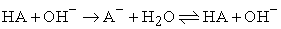(1)

and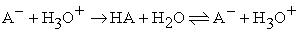(2)

wheredenotes the conjugate base for some weak acid HA.

We begin by considering the relationship between pH and pOH. For the purposes of this article, we assume the general definition of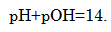(3)

Thus, if the pH of a solution decreases by some amount Δ, the pOH of that same solution will increase by that same amount Δ. On account of eq 3, we can generalize the buffer capacity definition to the following:

Definition 1 The buffer capacity of a solution is the amount (in mol) of strong acid or strong base that must be added to one liter of solution to change either the pH or pOH by one unit.

To begin the derivation, consider the Henderson-Hasselbalch representation for eqs 1 and 2: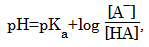(4)

whererepresents the acidic strength of HA.  Because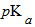is a constant value—one unique to HA—the only term in eq 4 that could cause a change in pH (or pOH) by ±1 unit must be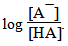. Let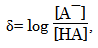(5)

thenBefore proceeding, note the definitions below.

Definition 2 We title a buffer solution as an imperfect buffer solution whenever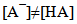and a perfect buffer solution wheneverDefinition 3 If the buffer capacity of a solution is being tested via strong base, we title the capacity as the base-buffer capacity. If instead the solution is being tested via strong acid, we title the capacity as the acid-buffer capacity.

We being by considering the addition of a strong base to an imperfect buffer solution (eq 1). As shown in eqs 1 and 2, the stoichiometric ratio of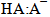is 1:1. Thus, if α mol of HA reacts completely with α mol of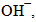then α mol of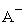will be produced (and eventually settled to equilibrium). Let Δ denote some arbitrary change in δ after α mol of HA have reacted. Then,(6)

Letdenote the base-buffer capacity for eq 1.{1} Then,and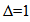because bases attempt to increase pH. We then obtain the formula

Identically,Solving for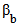producesSubstituting in δ (eq 5) and reformulating yields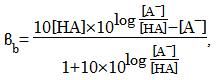which simplifies to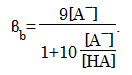Finally, multiplying both the numerator and denominator by [HA] yields the imperfect base-buffer capacity formula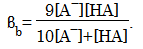(7)

In other words, the maximum amount of base (in mol) a one liter solution ofbuffer can withstand before experiencing a change in pH or pOH by one unit is given by eq 7.

In continuation, consider eq 2. Here a strong base is being added to the buffer solution. Let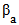denote the acid-buffer capacity for an imperfect buffer solution. To derive a suitable formula for, refer to eq 6. Because acids attempt to decrease pH, Δ= −1. Moreover, because the addition ofmol of strong acid will decrease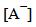and increase [HA] by(opposite to adding strong base),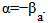Thus, utilizing eq 6, we acquire the formula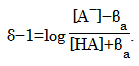Equivalently,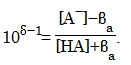We findSubstituting in δ and reformulating yieldsMultiplying both the numerator and denominator by 10[HA] produces the imperfect acid-buffer capacity formula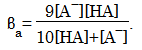(8)

Comparing eqs 7 and 8, we find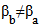for all δ≠0. This occurs because the buffer is imperfect. If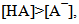then we would expect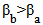because the buffer would have greater resistance to strong bases. If, however, the inverse were true, we would expectbecause the buffer would have greater resistance to strong acids.

We now consider a perfect buffer solution. Let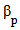denote the buffer capacity for a perfect buffer. Because a perfect buffer impliesδ=0. We find, utilizing eqs 7 and 8, a perfect buffer’s capacity—in terms of [HA]—to simply be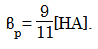(9)

Identically, in terms of(10)

Eqs 9 and 10 yield a very trivial, yet quite surprising result. Both state that the maximum amount of strong acid or base that can be added to a perfect buffer solution, before a change of either one pH or pOH unit occurs, is simply the product of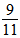and the concentration of either HA or A.

### 3. Limitations and Assumptions

Throughout this article we have assumed a simple buffer solution—that is, a buffer with only one weak acid or base and its corresponding conjugate. If multiple weak acids or bases (and their corresponding conjugates) are present, the methods in this paper will not yield proper results. Further, we have assumed that polyprotic acids and bases do not introduce an additional level of acidity or basicity, respectively. Realistically, however, if the buffer capacity of a solution is being tested via a strong, polyprotic acid or base, it is likely the capacity will come out relatively low. This will occur because the additional level of acidity or basicity will cause a faster change in pH (or pOH) in comparison to a strong, monoprotic acid or base.

### 4. Implications and Conclusions

In this article, we introduced a new buffer capacity definition and derivation intended for entry-level chemistry courses. The derivation provides a more understandable notion on buffer capacity by utilizing one of the most fundamental equations in introductory acid-base chemistry: the Henderson-Hasselbalch equation. We believe this method will grant more intuition on buffer capacity than the current, formal definition—thus allowing students to better understand from where their computational results evolve.

### Notes

The authors declare no competing financial interests.

### Acknowledgements

1. For emphasis,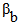is the amount (in mol) of OH being added to eq 1 to change either the pH or pOH by one unit.
  Brown, et al. Chemistry: The Central Science. 11th ed. Upper Saddle River, New Jersey: Pearson/Prentice Hall, 2008.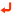In article  Sandell, E.B.; West, T.S. Recommended Nomenclature for Titrimetric Analysis. Pure Appl. Chem. 1969, 18 (3), 430.In article View Article  Hulanicki, A. Reaction of Acids and Bases in Analytical Chemistry. PWN & Ellis Horwood, 1987.In article  Po, H.N.; Senozan, N.M. The Henderson-Hasselbalch Equation: Its History and Limitations. J. Chem. Educ. 2001, 78 (11), 1499.In article View Article